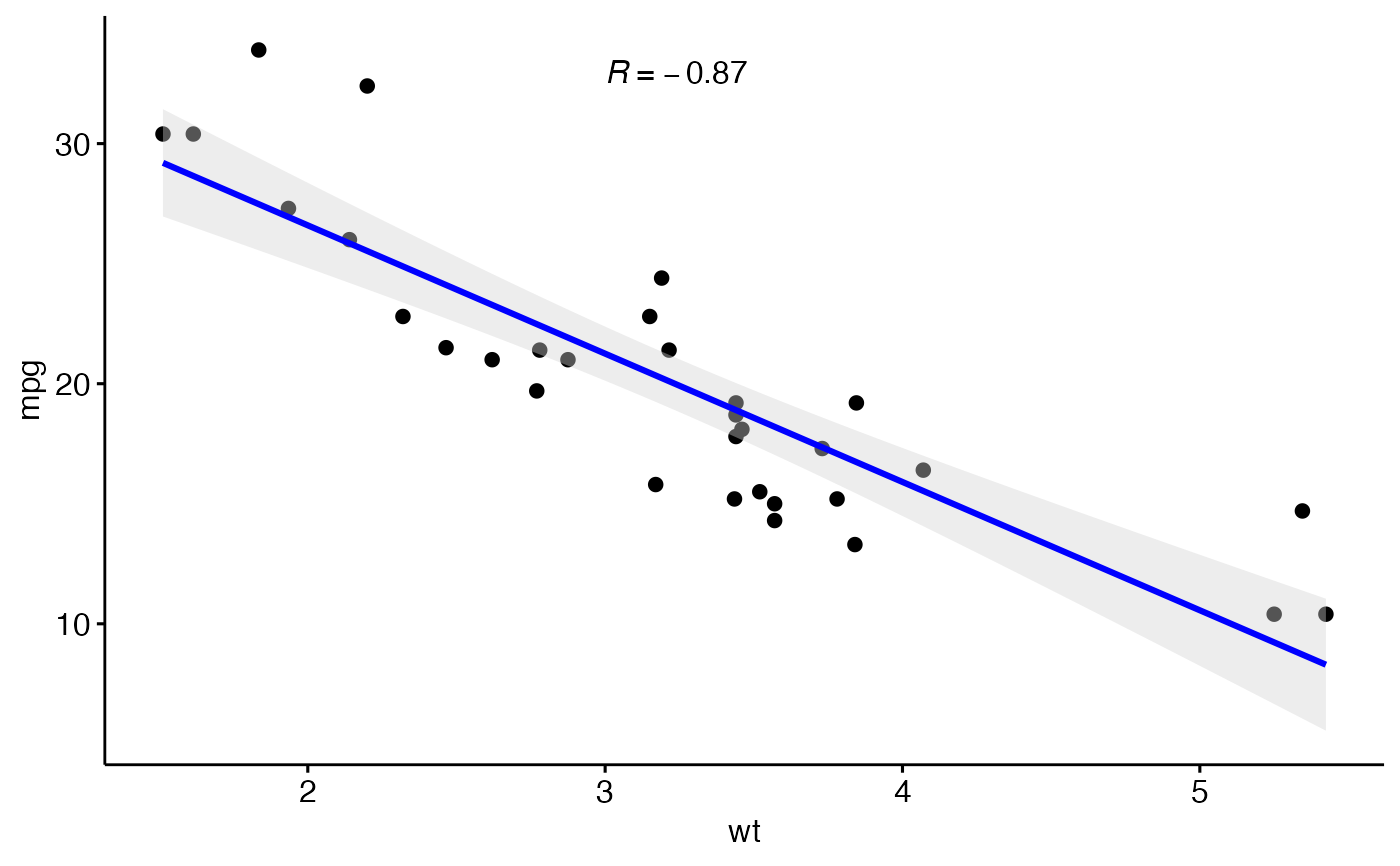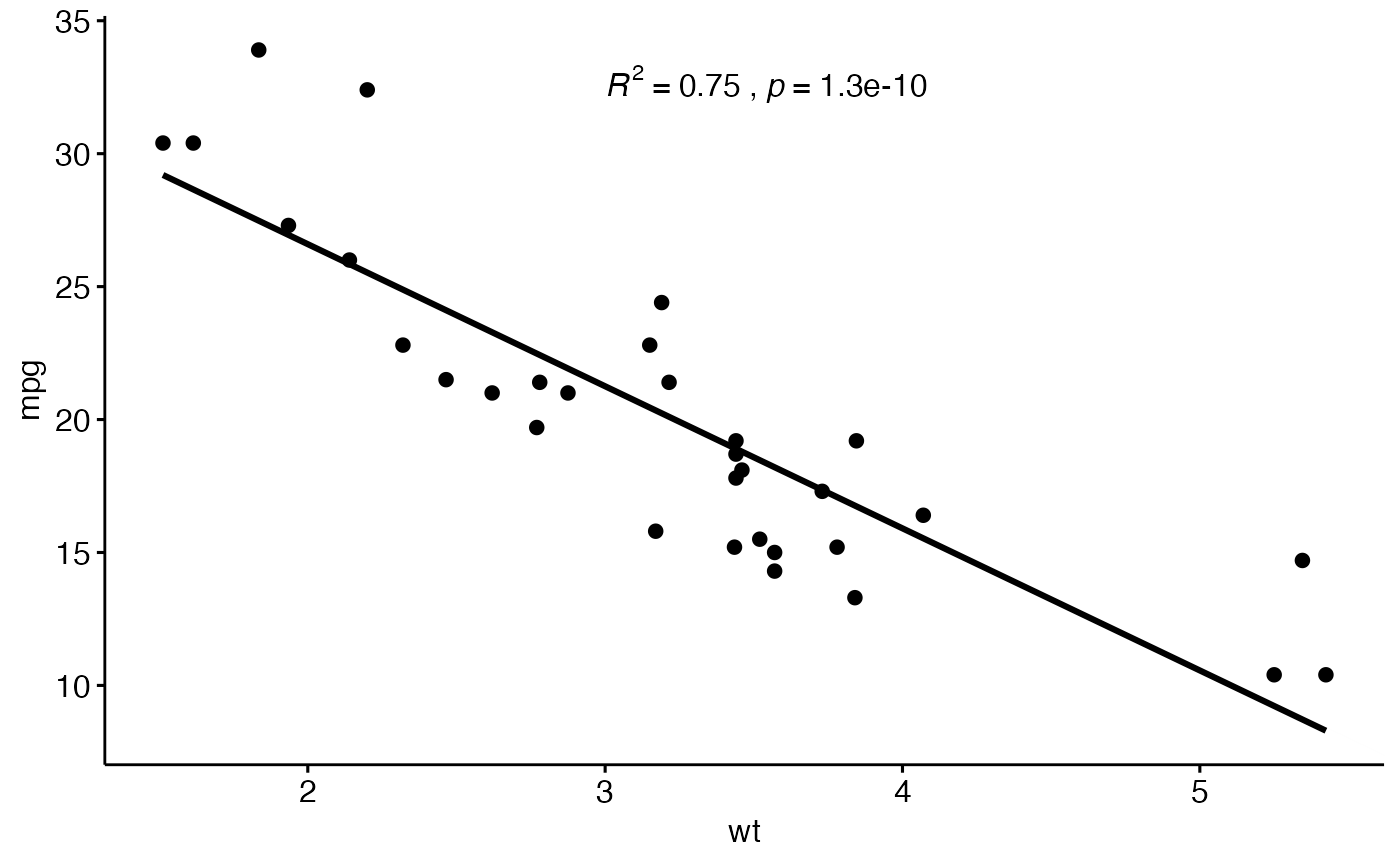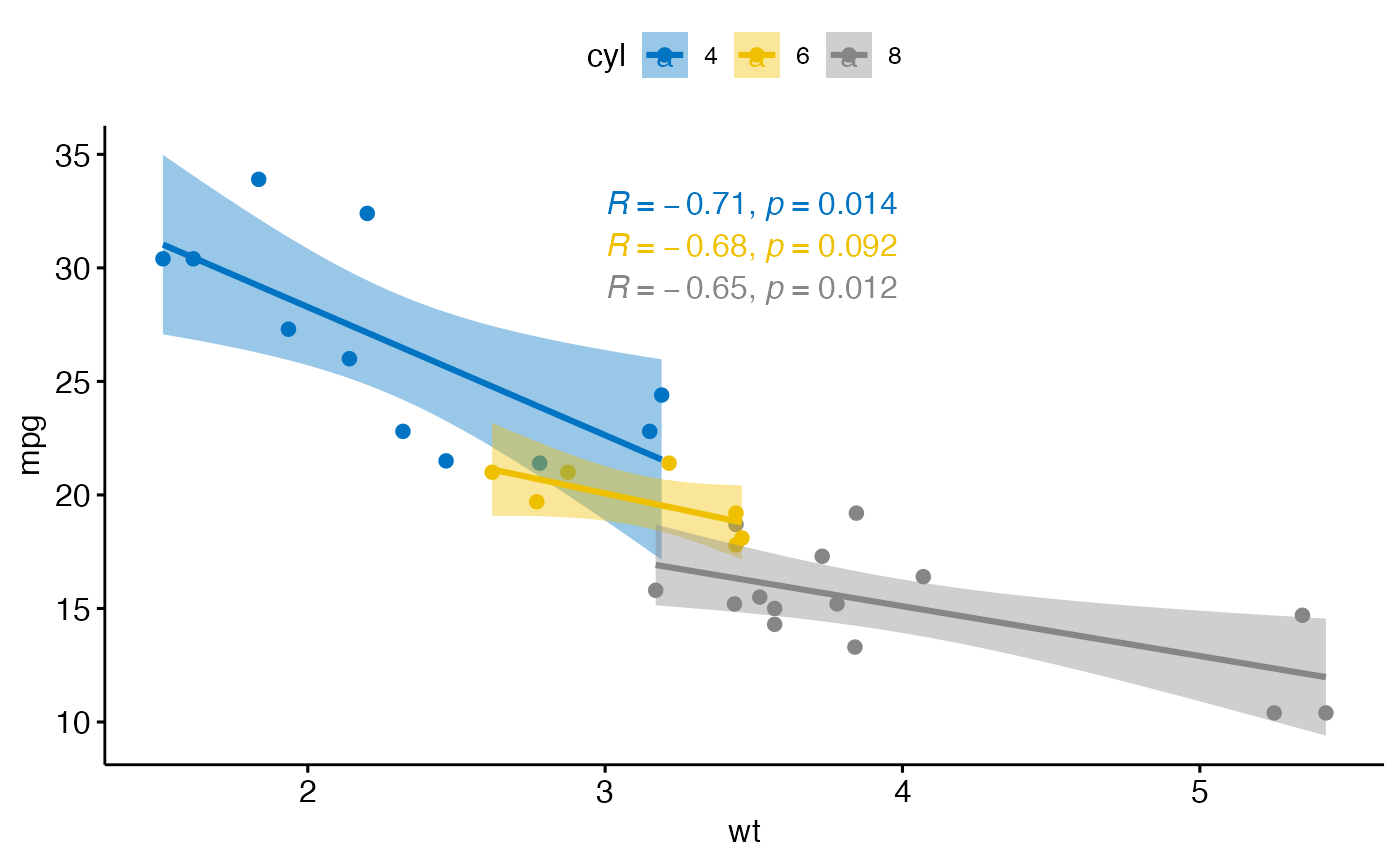Add correlation coefficients with p-values to a scatter plot. Can be also used to add R2.

stat_cor(
mapping = NULL,
data = NULL,
method = "pearson",
alternative = "two.sided",
cor.coef.name = c("R", "rho", "tau"),
label.sep = ", ",
label.x.npc = "left",
label.y.npc = "top",
label.x = NULL,
label.y = NULL,
output.type = "expression",
digits = 2,
r.digits = digits,
p.digits = digits,
r.accuracy = NULL,
p.accuracy = NULL,
geom = "text",
position = "identity",
na.rm = FALSE,
show.legend = NA,
inherit.aes = TRUE,
...
)

## Arguments

mapping

Set of aesthetic mappings created by aes(). If specified and inherit.aes = TRUE (the default), it is combined with the default mapping at the top level of the plot. You must supply mapping if there is no plot mapping.

data

The data to be displayed in this layer. There are three options:

If NULL, the default, the data is inherited from the plot data as specified in the call to ggplot().

A data.frame, or other object, will override the plot data. All objects will be fortified to produce a data frame. See fortify() for which variables will be created.

A function will be called with a single argument, the plot data. The return value must be a data.frame, and will be used as the layer data. A function can be created from a formula (e.g. ~ head(.x, 10)).

method

a character string indicating which correlation coefficient (or covariance) is to be computed. One of "pearson" (default), "kendall", or "spearman".

alternative

a character string specifying the alternative hypothesis, must be one of "two.sided" (default), "greater" or "less". You can specify just the initial letter.

cor.coef.name

character. Can be one of "R" (pearson coef), "rho" (spearman coef) and "tau" (kendall coef). Uppercase and lowercase are allowed.

label.sep

a character string to separate the terms. Default is ", ", to separate the correlation coefficient and the p.value.

label.x.npc, label.y.npc

can be numeric or character vector of the same length as the number of groups and/or panels. If too short they will be recycled.

• If numeric, value should be between 0 and 1. Coordinates to be used for positioning the label, expressed in "normalized parent coordinates".

• If character, allowed values include: i) one of c('right', 'left', 'center', 'centre', 'middle') for x-axis; ii) and one of c( 'bottom', 'top', 'center', 'centre', 'middle') for y-axis.

If too short they will be recycled.

label.x, label.y

numeric Coordinates (in data units) to be used for absolute positioning of the label. If too short they will be recycled.

output.type

character One of "expression", "latex", "tex" or "text".

digits, r.digits, p.digits

integer indicating the number of decimal places (round) or significant digits (signif) to be used for the correlation coefficient and the p-value, respectively..

r.accuracy

a real value specifying the number of decimal places of precision for the correlation coefficient. Default is NULL. Use (e.g.) 0.01 to show 2 decimal places of precision. If specified, then r.digits is ignored.

p.accuracy

a real value specifying the number of decimal places of precision for the p-value. Default is NULL. Use (e.g.) 0.0001 to show 4 decimal places of precision. If specified, then p.digits is ignored.

geom

The geometric object to use to display the data, either as a ggproto Geom subclass or as a string naming the geom stripped of the geom_ prefix (e.g. "point" rather than "geom_point")

position

Position adjustment, either as a string naming the adjustment (e.g. "jitter" to use position_jitter), or the result of a call to a position adjustment function. Use the latter if you need to change the settings of the adjustment.

na.rm

If FALSE (the default), removes missing values with a warning. If TRUE silently removes missing values.

show.legend

logical. Should this layer be included in the legends? NA, the default, includes if any aesthetics are mapped. FALSE never includes, and TRUE always includes. It can also be a named logical vector to finely select the aesthetics to display.

inherit.aes

If FALSE, overrides the default aesthetics, rather than combining with them. This is most useful for helper functions that define both data and aesthetics and shouldn't inherit behaviour from the default plot specification, e.g. borders().

...

other arguments to pass to geom_text or geom_label.

## Computed variables

r

correlation coefficient

rr

correlation coefficient squared

r.label

formatted label for the correlation coefficient

rr.label

formatted label for the squared correlation coefficient

p.label

label for the p-value

label

default labeldisplayed by stat_cor()

ggscatter

## Examples

# Load data
data("mtcars")
df <- mtcars
df$cyl <- as.factor(df$cyl)

# Scatter plot with correlation coefficient
#:::::::::::::::::::::::::::::::::::::::::::::::::
sp <- ggscatter(df, x = "wt", y = "mpg",
add.params = list(color = "blue", fill = "lightgray"), # Customize reg. line
conf.int = TRUE # Add confidence interval
)
sp + stat_cor(method = "pearson", label.x = 3, label.y = 30)# Specify the number of decimal places of precision for p and r
# Using 3 decimal places for the p-value and
# 2 decimal places for the correlation coefficient (r)
sp + stat_cor(p.accuracy = 0.001, r.accuracy = 0.01)# Show only the r.label but not the p.label
sp + stat_cor(aes(label = ..r.label..), label.x = 3)
#> Warning: The dot-dot notation (..r.label..) was deprecated in ggplot2 3.4.0.
#> ℹ Please use after_stat(r.label) instead.
#> ℹ The deprecated feature was likely used in the ggpubr package.
#>   Please report the issue at <https://github.com/kassambara/ggpubr/issues>.# Use R2 instead of R
ggscatter(df, x = "wt", y = "mpg", add = "reg.line") +
stat_cor(
aes(label = paste(..rr.label.., ..p.label.., sep = "~,~")),
label.x = 3
)# Color by groups and facet
#::::::::::::::::::::::::::::::::::::::::::::::::::::
sp <- ggscatter(df, x = "wt", y = "mpg",
color = "cyl", palette = "jco",
add = "reg.line", conf.int = TRUE)
sp + stat_cor(aes(color = cyl), label.x = 3)